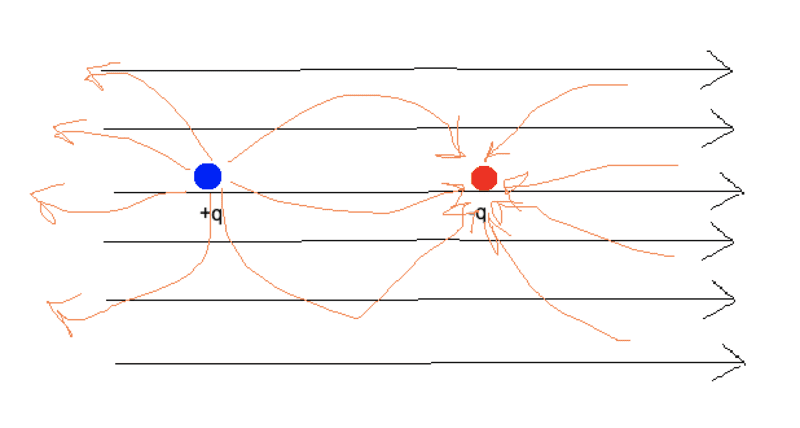# Will this dipole rotate or change position?

• I
annamal
Will this dipole rotate or change position? The external electric field is in black. Two charges with their electric fields are drawn in orange.Homework Helper
Gold Member
Calculate the net force on the dipole and the net torque (with respect to the center of the dipole) , you will find them both zero.

Another way to solve this, is via the concept of dipole moment. If the dipole moment is parallel to the external Electric Field then the dipole is in stable position.

Gold Member
So, the e-field arrows go from + charges (potential, really) towards minus. This will exert a coulomb force to push/pull the charges. But, it's stable like a pencil standing on it's point (really, an unstable equilibrium) any perturbation in alignment, which will always happen somehow, will create a rotational force to make them switch positions. Then it really will be stable (a stable equilibrium, once things settle down), but with the charges separated a bit more than if there was no field. The concept is simple, the + charge wants to move with the arrows, the minus charge wants to move the other way.

•berkeman
annamal
This confuses me a bit because the external electric field in black is as though we placed a positive charge to the very left of the image, right next to the blue +q, which means, the blue +q should be repelled and move away with the red -q moving forward.

Mentor
which means, the blue +q should be repelled and move away with the red -q moving forward.
That's what @DaveE just said. And if the two charges are held rigidly at their current separation (hence, a diople), they will be rotated by those forces until they align with the field. But since the E field is constant in magnitude in the area that you show, what is the "Net" force on the two charges overall?

annamal
That's what @DaveE just said. And if the two charges are held rigidly at their current separation (hence, a diople), they will be rotated by those forces until they align with the field. But since the E field is constant in magnitude in the area that you show, what is the "Net" force on the two charges overall?
Shouldn't it be 0 in this unstable equilibrium position?

Mentor
Shouldn't what be zero? The net force and torque? I suppose so, but it's a very unstable equilibrium as drawn, and will experience a torque fairly soon that flips it into the stable equilibrium position (after a period of oscillations that will depend on any damping that is present).

•DaveE
annamal
Shouldn't what be zero? The net force and torque? I suppose so, but it's a very unstable equilibrium as drawn, and will experience a torque fairly soon that flips it into the stable equilibrium position (after a period of oscillations that will depend on any damping that is present).
You asked about the net force. The net force should be 0.

Mentor
You asked about the net force. The net force should be 0.
Correct, but not the net torque. You were asking about how the dipole would move in this situation.